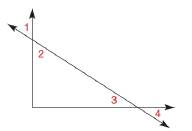Chapter 1.6, Problem 11E### Elementary Geometry for College St...

6th Edition
Daniel C. Alexander + 1 other
ISBN: 9781285195698

#### Solutions

Chapter
Section### Elementary Geometry for College St...

6th Edition
Daniel C. Alexander + 1 other
ISBN: 9781285195698
Textbook Problem
5 views

# In Exercise 11 and 12, provide the missing statements and reasons. Given: ∠ s 1 and 3 are complementary ∠ s 2 and 3 are complementary Prove: ∠ 1 ≅ ∠ 2 PROOF Statements Reasons 1. ∠ s 1 and 3 are complementary ∠ s 2 and 3 are complementary 1. ? 2. m ∠ 1 + m ∠ 3 = 90 ; m ∠ 2 + m ∠ 3 = 90 2. The sum of the measures of complementary ∠ s is 90 3. m ∠ 1 + m ∠ 3 = m ∠ 2 + m ∠ 3 3. ? 4. ? 4. Subtraction Property of Equality 5. ? 5. If two ∠ s are = in measure, they are ≅To determine

To find:

The missing statements and reasons.

Explanation

Given:

s 1 and 3 are complementary

s 2 and 3 are complementary

The given figure is,

Figure (1)

And,

The given table is shown below.

 PROOF Statements Reasons 1. ∠s 1 and 3 are complementary ∠s 2 and 3 are complementary 1. ? 2. m∠1+m∠3=90°;m∠2+m∠3=90° 2. The sum of the measures of complementary ∠s is 90° 3. m∠1+m∠3=m∠2+m∠3 3. ? 4. ? 4. Subtraction Property of Equality 5. ? 5. If two ∠s are = in measure, they are ≅

Approach:

1. The perpendicular lines are two lines that meet to form congruent adjacent angles.

2. If two lines are perpendicular, then they meet to form right angles.

3. If two angles are congruent, their measures are equal.

4. The symmetric property for the congruence of angles is satisfied, If 12, then 21.

5. If the sum of the measures of two angles is 90, then the angles are complementary.

Calculation:

The first statements of the proof are “s 1 and 3 are complementary” and “s 2 and 3 are complementary” So, these are the given conditions.

The reason for the first statements is “Given”

### Still sussing out bartleby?

Check out a sample textbook solution.

See a sample solution

#### The Solution to Your Study Problems

Bartleby provides explanations to thousands of textbook problems written by our experts, many with advanced degrees!

Get Started

#### [1, 4)

Applied Calculus for the Managerial, Life, and Social Sciences: A Brief Approach

#### In Problems 29 and 30, use TRACE or TABLE to evaluate the function.

Mathematical Applications for the Management, Life, and Social Sciences

#### The graph of x = cos t, y = sin2 t is:

Study Guide for Stewart's Single Variable Calculus: Early Transcendentals, 8th